# Plus Two Physics Chapter Wise Questions and Answers Chapter 10 Wave Optic

Students can Download Chapter 10 Wave Optic Questions and Answers, Plus Two Physics Chapter Wise Questions and Answers helps you to revise the complete Kerala State Syllabus and score more marks in your examinations.

## Kerala Plus Two Physics Chapter Wise Questions and Answers Chapter 10 Wave Optic

### Plus Two Physics Wave Optic NCERT Text Book Questions and Answers

Question 1.
What is the shape of the wavefront in each of the following cases:

1. Light diverging from a point source.
2. Light emerging out of a convex lens when a point source is placed at its focus.
3. The portion of the wavefront of light from a distant star intercepted by the earth.

1. It is spherical wavefront,
2. It is plane wavefront.
3. Plane wavefront (a small area on the surface of a large sphere is nearly planar).Question 2.
What is the Brewster angle for to glass transition? (refractive index glass = 1.5).
Given µ = 1.5, θ = ?
Since µ = tan θ
∴ tan θ = 1.5
or θ = tan-1 1.5 = 56.3°.

Question 3.
Light of wavelength 5000 Å falls on a reflecting surface. What are the wavelength and frequency of the reflected light? For what angle of incidence is the reflected ray normal to the incident ray?
Given λ = 5000 Å = 5 × 10-7m
The wavelength and frequency of reflected light remains same.
∴ Wavelength of reflected light,
λ = 5000 Å.
Frequency of reflected light,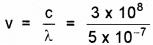= 6 × 1014HZ
The reflected ray is normal to incident if angle of incidence i = 45°.Question 4.
Estimate the distance for which ray optics is good approximation for an aperture of 4 mm and wavelength 400 nm.
Given a = 4mm = 4 × 10-3m.
λ = 400 nm = 4 × 10-7m
ZF = ?
∴ The minimum distance a beam of light has to travel before its deviation from straight line path becomes significant is called Fresnel distance ZF
∴ ZF = $$\frac{a^{2}}{\lambda}=\frac{16 \times 10^{-6}}{4 \times 10^{-7}}$$ = 40 m.

### Plus Two Physics Wave Optic One Mark Questions and Answers

Question 1.
The reddish appearance of the sun at sunrise and sunset is due to
(a) The scattering of light
(b) The polarisation of light
(c) The colour of the sun
(d) The colour of the sky
(a) The scattering of light
Explanation: The reddish appearance of the sun at sunrise and sunset is due to the scattering of light.Question 2.
Angle between the plane of vibration and plane of polarization is
(a) 30°
(b) 90°
(c) 60°
(d) 70°
(b) 90°
Explanation: Angle between the plane of vibration and plane of polarization is 90°.

Question 3.
If yellow light emitted by sodium lamp in Young’s double-slit experiment is replaced by monochromatic blue of light of the same intensity
(a) fringe width will decrease
(b) fringe width will increase
(c) fringe width will remain unchanged
(d) fringes will becomes less intense
(a) fringe width will decrease
Explanation: As β = $$\frac{\lambda D}{d}$$ and λb < λγ
∴ Fringe width p will decrease.Question 4.
A Young’s double-slit experiment uses a monochromatic source. The shape of the interference fringes formed on a screen
(a) straight line
(b) parabola
(c) hyperbola
(d) circle
(a) straight line
Explanation: Straight line fringes are formed on screen.

Question 5.
Find the odd one and justify interference, diffraction, polarisation.
Polarisation, because polarisation is possible only for transverse wave. So all other phenomenon are due to super position of waves.

Question 6.
State Malus law related to the intensity of light transmitted through the analyzer.
The Malus law states that the intensity of the polarized light transmitted varies as the square of the cosine of the angle between the plane of transmission of the analyser and plane of polarizer.Question 7.
What is the minimum thickness of a soap film needed for constructive interference in reflected light, if the light incident on the film is 750 nm? Assume that the refractive index for the film is µ = 1.33.
(a) 282 nm
(b) 70.5 nm
(c) 141 nm
(d) 387 nm
(c) 141 nm
Explanation: Here, 2µt = $$\frac{\lambda}{2}$$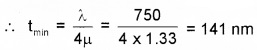Question 8.
Young’s experiment is performed with light of wavelength 6000 Å wherein 16 fringes occupy a certain region on the screen’. If 24 fringes occupy the same region with another light, of wavelength λ, then λ is
(a) 6000 Å
(b) 4500 Å
(c) 5000 Å
(d) 4000 Å
(d) 4000 Å
Explanation: n1λ1 = n2λ2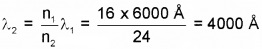### Plus Two Physics Wave Optic Two Mark Questions and Answers

Question 1.
Name the following wavefronts according to its nature.

1. Wave front due to point source.
2. Wave front due to fluorescent lamp
3. Emergent wavefront from a concave lens.
4. Emergent wavefront from a prism, when plane is incident on other face.

1. Spherical wave front
2. Cylindrical wavefront
3. Diverging wavefront
4. Plane wavefrontQuestion 2.
Two coherent sources have intensities in the ratio 25: 16. Find the ratio of intensities of maxima to minima after the superposition of waves from the two source.
I1 = a12 = 25, a1 = 5
I2 = a22 = 16
a2 = 4
Maximum intensity Imax = (a1 + a2)2 = (5 + 4)2 = 81
Minimum intensity Imin = (5 – 4)2 = 1

### Plus Two Physics Wave Optic Three Mark Questions and Answers

Question 1.
Fill in the blanks in three columns.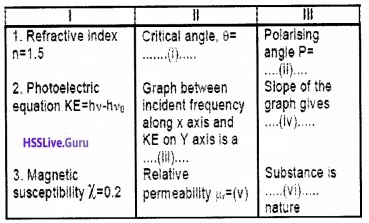(i) θ = 42°
(ii) P = 57°
(iii) Straight line
(iv) Planks constant
(v) µr = 1.2
(vi) Paramagnetic.Question 2.
Match the following suitably.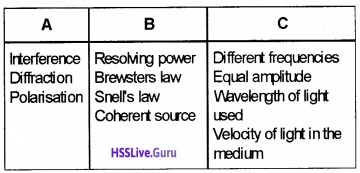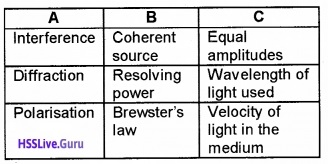Question 3.
Match the following

 A B 1. Colour of sky 1.  Interference 2. Rainbow 2. scattering 3. different colours seen in soap bubbles 3. Dispersion 4. Diffraction 5. Coherence 6. Looming

 A B 1. Colour of sky 2. Rainbow 3. different colours seen in soap bubbles 2. scattering 3. Dispersion 1. InterferenceQuestion 4.
A plane wave front is entering a lens is given in the figure

1. What is meant by a wave front
2. What are different types of wave fronts
3. Complete the diagram and draw the refracted wave front.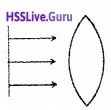1. Locus of all points having same phase of vibration is called wavefront.
2. Spherical wavefront, cylindrical wavefront plane wave front
3. Wave frond through a thin convex lens:Consider a plane wave passing through a thin convex lens. The central part of the incident plane wave travels through the thickest portion of lens. Hence central part get delayed. As a result the emerging wavefrond has a depression at the centre. Therefore the wave front becomes spherical and converges to a point F.

### Plus Two Physics Wave Optic Four Mark Questions and Answers

Question 1.
Thomas young successfully conducted double-slit experiment and explained the interference phenomenon using Huygens principle.

1. State Huygens wave theory.
2. State Huygens principle arrive at Snell’s law of refraction.
3. In the word of Hyugens “Light propagates as longitudinal waves” comment on the above statement.

1. According to Huygen’s principle

• Every point in a wavefront act as a source of secondary wavelets.
• The secondary wavelets travel with the same velocity as the original value.
• The envelope of all these secondary wavelets gives a new wavefront.

2. Huygen’s principle: According to Huygen’s principle:

• Every point in a wavefront acts as a source of secondary wavelets.
• The secondary wavelets travel with the same velocity as the original value.
• The envelope of all these secondary wavelets gives a new wavefront.

Refraction of a plane wave. (To prove Snell’s law):
AB is the incident wavefront and c1 is the velocity of the wavefront in the first medium. CD is the refracted wavefront and c2 is the velocity of the wavefront in the second medium. AC is a plane separating the two media.The time taken for the ray to travel from Pto R is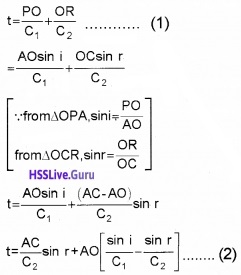O is an arbitrary point. Hence AO is a variable. But the time to travel a wavefront from AB to CD is constant. In order to satisfy this condition, the term containing AO in eq.(2) should be zero.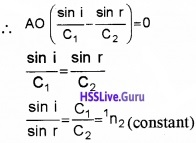where 1n2 is the refractive index of the second medium w.r.t. the first. This is the law of refraction.

3. Light wave cannot be longitudinal as it exhibit polarisation.Question 2.
A long narrow slit is illuminated by blue light and the diffraction pattern is obtained on a white screen.

1. How the width of bands change as the distance from the centre increases?
2. What happens to the width of pattern, if yellow light is used instead of blue light?
3. In a double-slit experiment, the slits are separated by 0.03cm and the screen is placed 1.5m away. The distance between the central fringe and the fourth bright fringe is 1 cm. Determine the wavelength of light used in the experiment.

1. Width of bands decreases from the centre of the bands

2. The pattern expands or bandwidth increases

3. xn = $$\frac{\mathrm{n} \lambda \mathrm{D}}{\mathrm{d}}$$
d = 0.03cm = 0.03 × 10-2 m
D = 1.5m, n = 4, xn = 1cm = 1 × 10-2m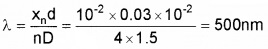Question 3.
Light of wavelength 589 nm is used to view an object under a microscope. The aperture of the objective has a diameter of 0.900cm.

1. What do you mean by limit of resolution of an optical instrument?
2. What is the limiting of resolution of the above microscope?
3. What happens to the limit of resolution if the objective is immersed in oil? Explain.

1. The minimum distance between two objects at which they can be observed as separated by an optical instrument is called the limit of resolution of the instrument.

2. Limit of resolution ∆θ = $$\frac{1.22 \lambda}{D}=\frac{1.22 \times 589 \times 10^{-9}}{0.9 \times 10^{-2}}$$

3. Decreases. Refractive index of oil is more than that of air. Hence wavelength of the light decreases.Question 4.
The law of refraction $$\frac{\sin i}{\sin r}=\frac{V_{1}}{V_{2}}$$

1. This law is called
2. Prove this law based on Huygiens wave theory.

1. Snells law

2. Refraction of a plane wave. (To prove Snell’s law):
AB is the incident wavefront and c1 is the velocity of the wavefront in the first medium. CD is the refracted wavefront and c2 is the velocity of the wavefront in the second medium. AC is a plane separating the two media.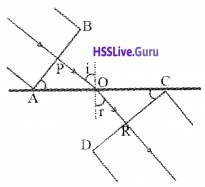The time taken for the ray to travel from Pto R is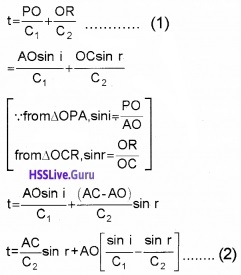O is an arbitrary point. Hence AO is a variable. But the time to travel a wavefront from AB to CD is constant. In order to satisfy this condition, the term containing AO in eq.(2) should be zero.where 1n2 is the refractive index of the second medium w.r.t. the first. This is the law of refraction.Question 5.
A long narrow slit is illuminated by blue light and the diffraction pattern is obtained on a white screen.

1. How the width of bands change as the distance from the centre increases? (1)
2. What happens to the width of pattern, if yellow light is used instead of blue light? (1)
3. In a double-slit experiment, the slits are separated by 0.03cm and the screen is placed 1.5m away. The distance between the central fringe and the fourth bright fringe is 1 cm. Determine the wavelength of light used in the experiment. (2)

1. Width of bands decreases from the centre of the bands

2. The pattern expands or bandwidth increases

3. xn = $$\frac{\mathrm{n} \lambda \mathrm{D}}{\mathrm{d}}$$
d = 0.03cm = 0.03 × 10-2 m
D = 1.5m, n = 4, xn = 1cm = 1 × 10-2m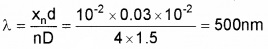Question 6.
Light of wavelength 589 nm is used to view an object under a microscope. The aperture of the objective has a diameter of 0.900cm.

1. What do you mean by limit of resolution of an optical instrument? (1)
2. What is the limiting of resolution of the above microscope? (1)
3. What happens to the limit of resolution if the objective is immersed in oil? Explain. (2)

1. The minimum distance between two objects at which they can be observed as separated by an optical instrument is called the limit of resolution of the instrument.

2. Limit of resolution3. Decreases. Refractive index of oil is more than that of air. Hence wavelength of the light decreases.Question 7.
A beam of light, with intensity I0, is passing through a polarizer and an analyzer as shown in figure.1. State Malus law related to the intensity of light transmitted through the analyzer. (1)

2. If 6 = 45°, what is the relation of the intensities of original light and transmitted light after passing through the analyzer? (2)

3. Which of the following waves can be polarized

• X-rays
• sound waves. Why? (1)

1. The Malus law states that the intensity of the polarized light transmitted varies as the square of the cosine of the angle between the plane of transmission of the analyser and plane of polarizer.

2.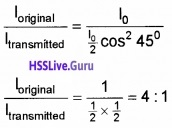3. X-rays. Because it is a transverse wave.

### Plus Two Physics Wave Optic Five Mark Questions and Answers

Question 1.
Consider a point source emitting waves uniformly in all directions.

1. Draw two wave fronts very near to the point source. (1)
2. Using Huygen’s principle, prove that angle of incidence is equal to angle of reflection. (3)
3. What is the shape of a plane wave front after passing through a thin convex lens? (1)

1.2.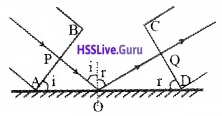AB is the incident wavefront and CD is the reflected wavefront, ‘i’ is the angle of incidence and r’ is the angle of reflection. Let c1 be the velocity of light in the medium. Let PO be the incident ray and OQ be the reflected ray.
The time taken for the ray to travel from P to Q isO is an arbitrary point. Hence AO is a variable. But the time to travel for a wave front from AB to CD is a constant. So eq.(2) should be independent of AO. i.e., the term containing AO in eq.(2) should be zero.
∴ $$\frac{A O}{C_{1}}$$(sin i – sin r) = 0
sin i – sin r = 0
sin i – sin r
i = r
This is the law of reflection.

3. Spherical wavefront.

Question 2.
A slit S is illuminated by a monochromatic suorce of light to give two coherent sources P1 and P2. These give a dark band at the point R on the screen as shown in figure.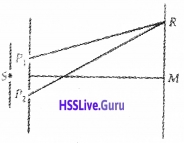1. Write the formula to find out bandwidth. (1)
2. What relationship must exist between the length P1R and P2R? (1)
3. Can interference fringes be produced by using two identical bulbs? (1)
4. If the distance between P1 and P2 is 1 mm and the screen is placed in 1m away, what is the fringe separation for a light of wavelength 5 × 10-7m?(2)

1. β = $$\frac{\lambda D}{d}$$

2. The condition for dark fringe is P2R – P1R = (2n -1)$$\frac{\lambda }{2}$$ where n = 1,2, 3, etc.

3. No. They are not coherent sources.

4.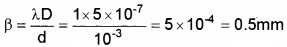Question 3.
In interference, when light energy superimpose with a phase difference 180°, darkness occurs.

1. Two sources which can give sustained interference pattern is said to be …….
2. Does interference phenomenon violate law of conservation of energy? Justify.
3. Modify the expression for bandwidth in terms of refractive index of medium between slit and screen.

1. Coherent sources.

2. No. In interference, only energy is redistributed.

3. We know refractive index n = $$\frac{c}{v}=\frac{\lambda}{\lambda_{1}}$$
λ1 = $$\frac{\lambda}{n}$$ substituting this in the expression for bandwidth $$\left(\beta=\frac{\lambda, \mathrm{D}}{\mathrm{d}}\right)$$
we get $$\beta=\frac{\lambda D}{n d}$$.Question 4.
Figure below shows a version of Young’s Experiment performed by directing a beam of electrons on a double slit. The screen reveals a pattern of bright and dark fringes similar to an interference pattern produced when a beam of light is used.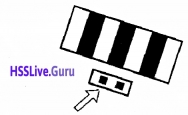1. Which property of electron is revealed in this observation.
2. If the electrons are accelerated by a p.d. of 54v, what is the value of wavelength associated with electrons.
3. In similar experiment, if the electron beam is re-placed by bullets fired from a gun, no interference pattern is observed. Give reason.

1. Dual nature or wave nature.

2.3. λ = $$\frac{\mathrm{h}}{\mathrm{mv}}$$. Since the mass ofthe bullet is very much greater than the mass of electron, the de Broglie wavelength is not appreciable.Question 5.
A. In young’s double-slit experiment two slits are illuminated by real monochromatic light source.

1. If one of the slits is closed, what will be the observation on the screen?
2. Arrive at an expression for bandwidth of interference fringes, when both the slits are open.
3. What happens to the bandwidths, if the experimental arrangement is immersed in water?

1. Single slit diffraction pattern.

2. Expression for band width: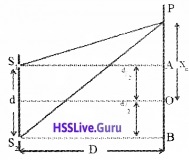S1 and S2 are two coherent sources having wave length λ. Let ‘d’ be the distance between two coherent sources. A screen is placed at a distance D from sources. ‘O’ is a point on the screen equidistant from S1 and S2.

Hence the path difference, S1O – S2O = 0
So at ‘O’ maximum brightness is obtained.
Let ‘P’ be the position of nth bright band at a distance xn from O. Draw S1A and S2B as shown in figure.
From the right angle ∆S1AP
we get, S1P2 = S1A2 + AP2
S1P2 = D2 + (Xn – d/2)2
= D2 + Xn2 – Xnd + $$\frac{\mathrm{d}^{4}}{4}$$
Similarly from ∆S2BP weget,S2P2 = S2B2 + BP2
S2P2 = D2 + (Xn + d/2)2S2P2 – S1P2 =2xnd (S2P + S1P)(S2P – S1P) = 2xnd
But S1P ≈ S2P ≈ D
∴ 2D(S2P – S1P) = 2xnd
i.e., path difference S2P – S1P = $$\frac{x_{n} d}{D}$$ ____(1)
But we know constructive interference takes place at P, So we can take
(S2P – S1P) = nλ
Hence eq(1) can be written asLet xn+1 be the distance of (n+1)th bright band from centre o, then we can write∴ band width, b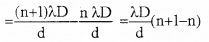β = $$\frac{\lambda \mathrm{D}}{\mathrm{d}}$$
This is the width of the bright band. It is the same for the dark band also.

3. Since wavelength decreases, the bandwidth decreases.Question 6.
Green light is incident at the Polarizing angle on a certain glass plate as shown in figure.1. What do you mean by polarizing angle?
2. Indicate the polarization components on the reflected and refracted rays, by arrows and dots.
3. Find the refractive index of glass.

1. The angle of incidence, at which incident light on a transparent medium, become completely plane polarized is known as polarizing angle.

2.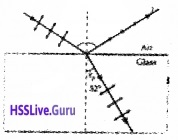3. Polarizing angle = 90° – 32° = 58°, Refractive Index, n = tan 58° = 1.6.Question 7.
Allow to lights rays to incident on a screen after passing through two slits.

1. Why light is passed through two slits.
2. Find the expression for fringe width
3. What happens to the pattern on the screen when the whole apparatus is dipped in water

1. Because light has wave nature. Two slits gives effect of coherent sources.

2. Expression for band width: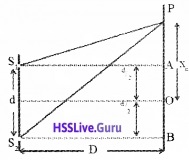S1 and S2 are two coherent sources having wave length λ. Let ‘d’ be the distance between two coherent sources. A screen is placed at a distance D from sources. ‘O’ is a point on the screen equidistant from S1 and S2.

Hence the path difference, S1O – S2O = 0
So at ‘O’ maximum brightness is obtained.
Let ‘P’ be the position of nth bright band at a distance xn from O. Draw S1A and S2B as shown in figure.
From the right angle ∆S1AP
we get, S1P2 = S1A2 + AP2
S1P2 = D2 + (Xn – d/2)2
= D2 + Xn2 – Xnd + $$\frac{\mathrm{d}^{4}}{4}$$
Similarly from ∆S2BP weget,
S2P2 = S2B2 + BP2
S2P2 = D2 + (Xn + d/2)2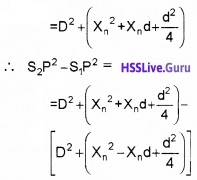S2P2 – S1P2 =2xnd (S2P + S1P)(S2P – S1P) = 2xnd
But S1P ≈ S2P ≈ D
∴ 2D(S2P – S1P) = 2xnd
i.e., path difference S2P – S1P = $$\frac{x_{n} d}{D}$$ ____(1)
But we know constructive interference takes place at P, So we can take
(S2P – S1P) = nλ
Hence eq(1) can be written as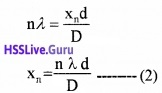Let xn+1 be the distance of (n+1)th bright band from centre o, then we can write∴ band width, b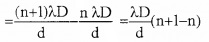β = $$\frac{\lambda \mathrm{D}}{\mathrm{d}}$$
This is the width of the bright band. It is the same for the dark band also.

3. The pattern shrinks (Band width decreases) if whole apparatus is dipped in water. (Because of high refractive index, velocity of light decreases. The wavelength also decreases and hence fringe width also reduce).Question 8.
The double slit in Young’s experiment is replaced by a single narrow slit (illuminated by a monochromatic source). Then,

1. How the pattern of bands on the screen differ from the pattern due to double slit? (1)
2. Derive an expression for the bandwidth of the central fringe. (3)
3. Draw a graph which shows the variation of intensity of light with distance. (1)

1. A broad pattern with a central bright region is seen. On both sides, there are alternate dark and bright regions of decreasing intensity.

2. Consider a point P, on the screen at which wavelets travelling in a direction making an angle θ with CO are brought to focus by the lens are shown in figure.These wavelets travel unequal distances in reaching the point P1. Hence these waves are not in phase. The wavelets from the points A and B reaching P1 are having a path difference, BP1 – AP1 = BN = a sinθ.

This path difference equals to λ. Then for each point in the upper half AC of the slit, there is a corresponding point in the lower half CB such that the wavelets from these two points reach at P1 with a path difference of λ/2.

These wavelets interfere destructively to make the intensity at P1 minimum. The point P1corresponds to first minima. Condition for first minima is a sinθ = λ.Thus in general, the minima will occur when the path difference = asinθ = λ where n = 1,2,3. Thus minima are formed on both sides of O, i.e. the central maxima. In between minima, other maxima called secondary maxima are formed. Secondary maxima will be at those points for which the path difference for the rays is asinθ = (2n + 1)$$\frac{\lambda}{2}$$.

The width of the central maximum is defined as the distance between the first minima on either side of the central maximum. For the first minimum, a sinθ = λ when θ is small sinθ = θ. i.e.a θ = λ.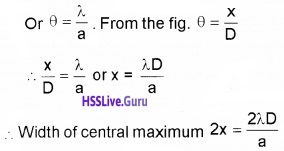3.Question 9.
A slit S is illuminated by a monochromatic suorce of light to give two coherent sources P1 and P2. These give a dark band at the point R on the screen as shown in figure.1. Write the formula to find out bandwidth, (1)
2. What relationship must exist between the length P1R and P21R? (1)
3. Can interference fringes be produced by using two identical bulbs? (1)
4. If the distance between P1 and P2 is 1 mm and the screen is placed in 1m away, what is the fringe separation for a light of wavelength 5 × 10-7m?(2)

1. β = $$\frac{\lambda D}{d}$$

2. The condition for dark fringe is P2R – P1R = (2n -1)$$\frac{\lambda }{2}$$ where n = 1,2, 3, etc.

3. No. They are not coherent sources.

4.Question 10.
The Photograph given below is obtained by passing a LASER beam on a pain of closely spaced slits.1. Identify this pattern.
2. Obtain an expression for bandwidth of the pattern.
3. In the double slit experiment using wavelength 5461 A0, the fringe width measured is 0.15mm. By keeping the same arrangement, the fringe width is measured for an unknown wavelength is 0.12mm. Find the unknown wave length.
4. If you change the LASER light from red to blue, what will happen to the space between the pattern shown in photograph. Justify.

1. Interference

2. Expression for band width: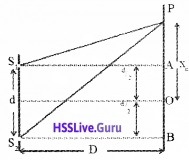S1 and S2 are two coherent sources having wave length λ. Let ‘d’ be the distance between two coherent sources. A screen is placed at a distance D from sources. ‘O’ is a point on the screen equidistant from S1 and S2.

Hence the path difference, S1O – S2O = 0
So at ‘O’ maximum brightness is obtained.
Let ‘P’ be the position of nth bright band at a distance xn from O. Draw S1A and S2B as shown in figure.
From the right angle ∆S1AP
we get, S1P2 = S1A2 + AP2
S1P2 = D2 + (Xn – d/2)2
= D2 + Xn2 – Xnd + $$\frac{\mathrm{d}^{4}}{4}$$
Similarly from ∆S2BP weget,
S2P2 = S2B2 + BP2
S2P2 = D2 + (Xn + d/2)2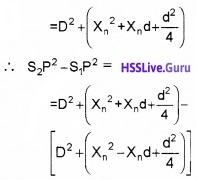S2P2 – S1P2 =2xnd (S2P + S1P)(S2P – S1P) = 2xnd
But S1P ≈ S2P ≈ D
∴ 2D(S2P – S1P) = 2xnd
i.e., path difference S2P – S1P = $$\frac{x_{n} d}{D}$$ ____(1)
But we know constructive interference takes place at P, So we can take
(S2P – S1P) = nλ
Hence eq(1) can be written asLet xn+1 be the distance of (n+1)th bright band from centre o, then we can write∴ band width, bβ = $$\frac{\lambda \mathrm{D}}{\mathrm{d}}$$
This is the width of the bright band. It is the same for the dark band also.

3.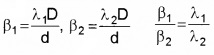λ1 = 5461 A° = 5461 × 10-10 m
β1 = 0.15mm = 0.15 × 10-3m
β2 = 0.12mm = 0.12 × 10-3m.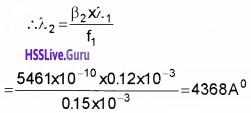4. Wave length of blue is less than that of red. Hence β decreases.Question 11.
According to a principle, at a particular point in a medium, the resultant displacement produced by a number of waves is the vector sum of the displacements produced by each of the waves.

1. Name of the principle.
2. Derive an expression for the bandwidth in Young’s double-slit experiment.

1. Superposition principle.

2. Expression for band width: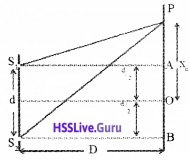S1 and S2 are two coherent sources having wave length λ. Let ‘d’ be the distance between two coherent sources. A screen is placed at a distance D from sources. ‘O’ is a point on the screen equidistant from S1 and S2.

Hence the path difference, S1O – S2O = 0
So at ‘O’ maximum brightness is obtained.
Let ‘P’ be the position of nth bright band at a distance xn from O. Draw S1A and S2B as shown in figure.
From the right angle ∆S1AP
we get, S1P2 = S1A2 + AP2
S1P2 = D2 + (Xn – d/2)2
= D2 + Xn2 – Xnd + $$\frac{\mathrm{d}^{4}}{4}$$
Similarly from ∆S2BP weget,
S2P2 = S2B2 + BP2
S2P2 = D2 + (Xn + d/2)2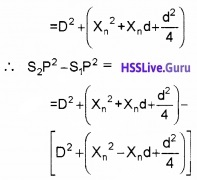S2P2 – S1P2 =2xnd (S2P + S1P)(S2P – S1P) = 2xnd
But S1P ≈ S2P ≈ D
∴ 2D(S2P – S1P) = 2xnd
i.e., path difference S2P – S1P = $$\frac{x_{n} d}{D}$$ ………1)
But we know constructive interference takes place at P, So we can take
(S2P – S1P) = nλ
Hence eq(1) can be written asLet xn+1 be the distance of (n+1)th bright band from centre o, then we can write∴ band width, bβ = $$\frac{\lambda \mathrm{D}}{\mathrm{d}}$$
This is the width of the bright band. It is the same for the dark band also.